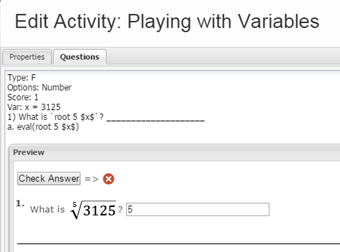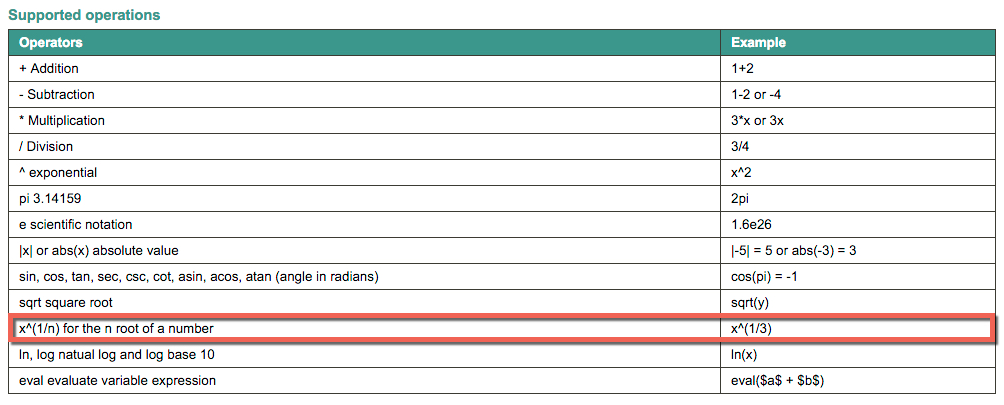## Math question about square roots, cubed roots, etc.

Follow

One of our math teachers was asking me today how to have Buzz calculate a 5th root of a number.  I know if you want to get a square root, you would use sqrt(\$x\$).  Is there an easy way to have Buzz calculate a cubed root or a fifth root?

Sort by

Flew, I would have to confirm with our developers (I'm no math genius), but I believe that the following expression is what you would want. This is a ASCII math expression:

``root y x``Please let me know if this does or does not meet the need. If it doesn't, please give me an example of what it is supposed to look like.

Thanks.

0

That helps for the first part, displaying the root in the question.  What do I put in the answer though to have Buzz calculate the root?

Type: F
Options: Number
Score: 1
Var: x = 3125
1) What is `root 5 \$x\$`?
a. What do I put here?

0

Type: F
Options: Number
Score: 1
Var: x = 3125
1) What is `root 5 \$x\$`?
a. eval(root 5 \$x\$)

0

I couldn't get that to work.0

Let me look further into it.

0

Flew, try this.

Type: F
Options: Number
Score: 1
Var: x = 3125
1) What is `root 5 \$x\$`? ____________________
a. eval(\$x\$^(1/5))

0

Thanks for getting that for us!

0

Great. I got that from the Supported Operations table from this documentation (third operation from the bottom). It will eventually be rolled over into the Community once we revamp authoring.

Here is a picture of the table:0